Sudoku solvers have been written in SAS using a variety of methods (e.g., the DATA step, PROC SQL, and PROC CLP). Surprisingly, SAS/IML appears to have been overlooked for this purpose. On a challenge from a coworker, I wrote this blog post to demonstrate the flexibility of SAS/IML in the context of solving Sudoku puzzles. This topic is split into two parts. Part 1 (this post) describes how Sudoku puzzles can be treated as exact cover problems and, in many cases, solved with simple logic. Part 2 describes how advanced Sudoku puzzles can be solved through a combination of the simple solver presented in part 1 and an efficient backtracking algorithm developed by Donald Knuth (which he labeled Algorithm X). Part 2 will be released in a separate post in the next two weeks. (Here is a link to Part 2)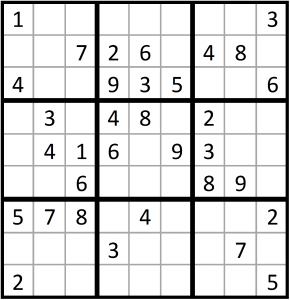Background

A Sudoku puzzle consists of a partially filled-in 9x9 grid. The grid contains 9 rows, 9 columns, and 9 3x3 boxes. Each cell must contain a single integer between 1 and 9. Each row, column, and box must contain every integer between 1 and 9.

Exact Cover

Before examining how Sudoku can be represented as an exact cover problem, let’s discuss exact cover problems generally. Consider a set containing the integers 1 through 9:  X = {1,2,3,4,5,6,7,8,9}. Now consider a collection of subsets of X, A-F.

A = {1,3,9}
B = {1,2,3}
C = {2,8,9}
D = {4,5,6}
E = {2,7,8}
F = {1,5,8}

Each subset contains some, but not all, of the numbers in X. The solution of an exact cover problem is the collection of subsets that represent every number in X exactly once. Because subsets A, D, and E represent every value in X once and only once, the subcollection {A,D,E} is said to be an exact cover of X.

A = {1,3,9}
D = {4,5,6}
E = {2,7,8}

This exact cover problem can be represented as a 6x9 indicator matrix: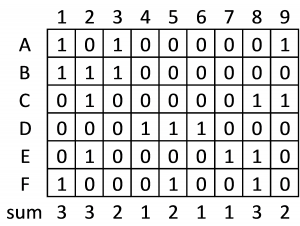Note that each row corresponds to a subset and each column corresponds to a number. Because subset A contains the numbers one, three, and nine, we set cells A1, A3, and A9 equal to one. Because subset A does not contain any other numbers, all other cells in row A are set to zero. We construct rows B through F in the same fashion. The column sums at the bottom of the table tell us the number of times that each number appears across the subsets.

Representing exact cover problems as matrices makes simple exact cover problems easy to solve. First, remember that each number must be included in the final collection of subsets. If a column has a sum of one, this means that the number represented by the column only appears in one of the subsets. Thus, we know that the subset containing the number must be included in the final collection of subsets.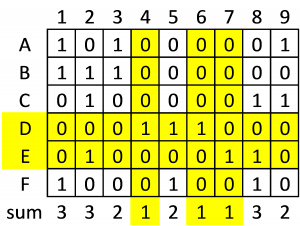In the current example, we can see that the columns corresponding to numbers 4 and 6 each have a sum of 1, as the two numbers appear only in subset D. Similarly, the column corresponding to number 7 has a sum of 1, as the number appears only in subset E. So, we know that subsets D and E must appear in the final collection of subsets.

Knowing that two subsets must appear in the matrix, we can now rule out some competing subsets. Subsets D and E must appear in the final collection, so any other subset containing a number that appears in subsets D or E cannot be included in the final collection. We can check for such conflicts by looking for columns where either D or E is equal to one and checking whether any other rows contain a one in the same column.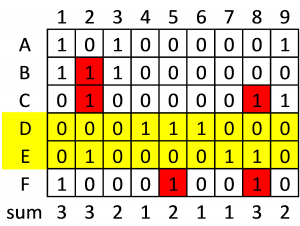We find that subsets B, C, and F conflict with subsets D and E. That is, subsets B and E both contain the number 2. Subsets C and E both contain numbers 2 and 8. Subset F contains the number 5, which is contained in D, and also 8, which is contained in E. Subsets B, C, and F can be effectively removed from consideration by setting all of the cells in their corresponding rows to zero. After setting the cells in rows B, C, and F to zero, we find that numbers 1, 3, and 9 are represented only in subset A (the associated column sums are 1). Thus, subset A must be included in the final collection.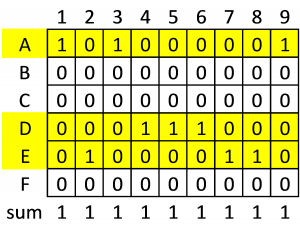Every column now has a sum of one. This means that every number is included once and only once in our final collection of subsets, {A,D,E}, and the subsets are therefore an exact cover of our set.

Sudoku as an Exact Cover Problem

To describe Sudoku as an exact cover problem we begin by translating the Sudoku grid into subsets. Specifically, we create a subset for every possible row/column/number combination, ignoring the information in the initial Sudoku grid. For example, subset r1c1n1 would correspond to row 1 column 1 containing a 1, subset r1c1n2 would correspond to row 1 column 1 containing a 2, etc. Since we have 9 rows, 9 columns, and 9 possible values per cell, the Sudoku grid is represented by 9x9x9 = 729 subsets. Thus, our exact cover matrix will contain 729 rows.

We next create elements for the subsets based on the Sudoku rules. Each row/column/number combination fulfills four Sudoku constraints. For example, r3c5n9 fulfills the following constraints: cell r3c5 must contain an integer; row 3 must contain the number 9; column 5 must contain the number 9; box 2 must contain the number 9. Each subset contains four elements, one for each constraint fulfilled by the subset.

Each cell must contain a single integer between 1 and 9.

This rule requires 81 constraints, one per cell. We represent each cell as a row/column pair, and assign each row/column/number subset an element for its corresponding row/column pair. Subsets r1c1n1, r1c1n2, …, r1c1n9 all contain r1c1, etc. The exact cover matrix will contain 81 columns corresponding to these cell constraints. The portion of the exact cover matrix corresponding to these constraints (with zeroes replaced by blanks for clarity) is: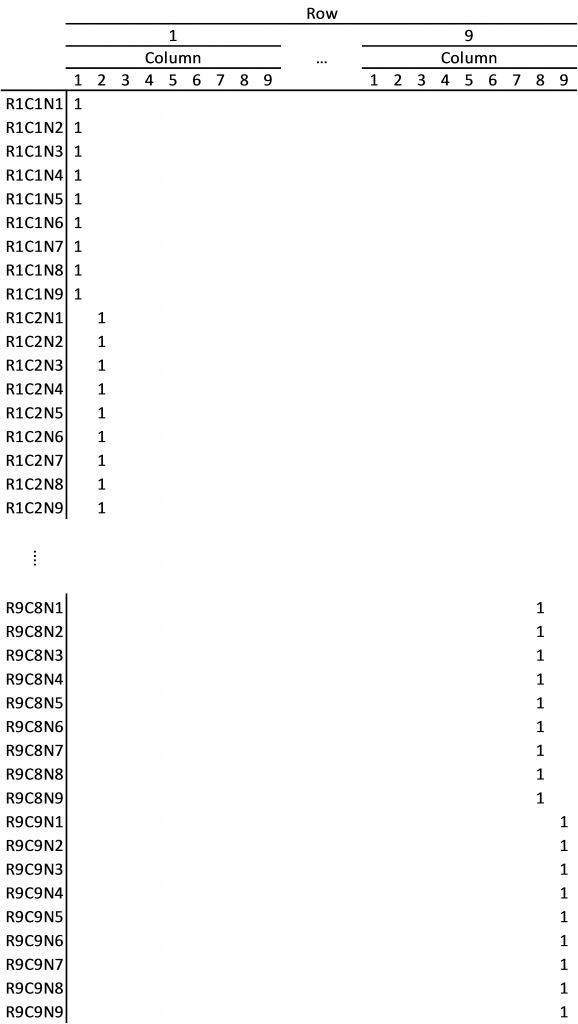Each row must contain every integer between 1 and 9.

This rule requires 81 constraints, one for every row/number combination. We assign each subset an element for its corresponding row/number pair. Subsets r1c1n1, r1c2n1, …, r1c9n1 all contain r1n1, etc. The exact cover matrix will contain 81 columns corresponding to these row/number constraints. The portion of the exact cover matrix corresponding to these constraints (with zeroes replaced by blanks for clarity) is: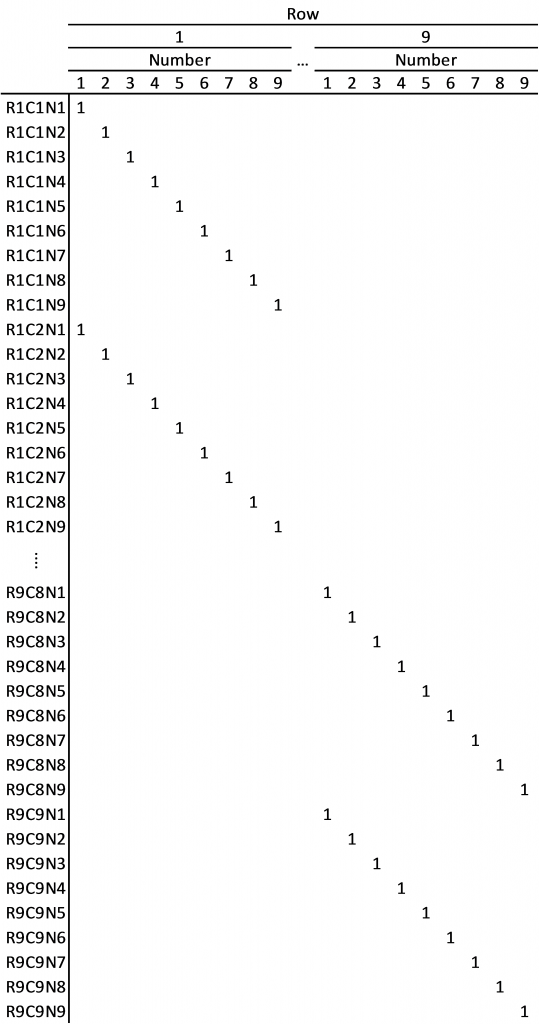Each column must contain every integer between 1 and 9.

This rule requires 81 constraints, one for every column/number combination. We assign each subset an element for its corresponding column/number pair. Subsets r1c1n1, r2c1n1, …, r9c1n1 all contain c1n1, etc. The exact cover matrix will contain 81 columns corresponding to these column/number constraints. The portion of the exact cover matrix corresponding to these constraints (with zeroes replaced by blanks for clarity) is:Each box must contain every integer between 1 and 9.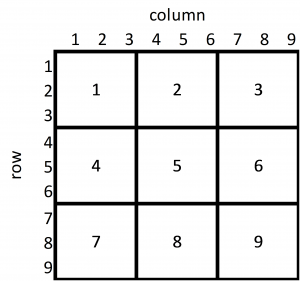This rule requires 81 constraints, one for every box/number combination. We assign each subset an element for its corresponding box/number pair. The boxes are numbered as shown in the figure on the right. Subsets r1c1n1, r1c2n1, …, r3c3n1 all contain b1n1, etc. The exact cover matrix will contain 81 columns corresponding to these box/number constraints. The portion of the exact cover matrix corresponding to these constraints (with zeroes replaced by blanks for clarity) is: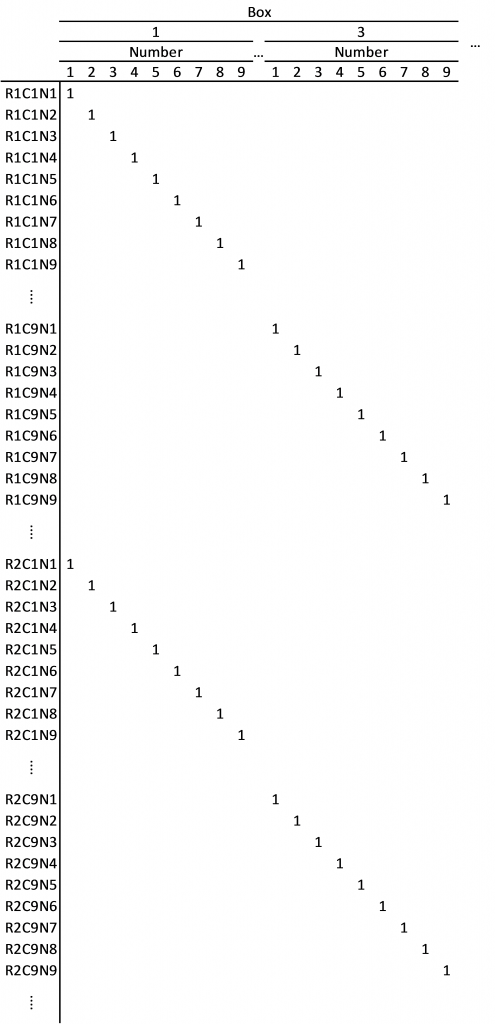The four 729x81 matrices can be horizontally concatenated to form the entire 729x324 Sudoku exact cover matrix. The entire matrix is available from Bob Hanson:

http://www.stolaf.edu/people/hansonr/sudoku/exactcovermatrix.htm

Enter Known Values

To update the exact cover matrix with information from the starting Sudoku grid we simply eliminate subsets that compete with the subsets represented in the initial matrix. A competing row is any row in the exact cover matrix that contains a 1 in the same column as a row that is known to be in the final subcollection (I’ll label this as a “selected” subset). For the starting Sudoku matrix at the beginning of this post, we see a 1 in row 1 column 1. This tells us that subset r1c1n1 must be selected. We must also select r1c9n3, r2c3n7, r2c4n2, etc. The cell constraint portion of the exact cover matrix shows us that subsets r1c1n2, r1c1n3, …, r1c1n9 all compete with subset r1c1n1 for fulfilling the r1c1 constraint. We set the values for those rows in the r1c1 column to zero.

Basic Sudoku Solver

The basic solver consists of a simple loop:

1. Select subsets. If only one subset fulfills a particular constraint (e.g., a 9 must be included in row 5 and r5c4n9 is the only remaining subset that fulfills this criterion), then select this subset. We know that a constraint can only be fulfilled by a single subset if the associated column sums to one, and the only non-zero row in the column must be selected.
2. Eliminate competitors. Changing any of the four non-zero values in a row to zero effectively eliminates that row. After selecting a subset, we examine all other columns associated with that subset and set every other row in these columns to zero.
3. Clean up matrix. Rows eliminated in step 2 can still contain non-zero values. In this step we check for rows that have sums between 1 and 3 and set every value in these rows to zero.

This loop runs until no more changes are made to the exact cover matrix. If every column sums to one, the puzzle is solved. If any column sums to zero, an impossible solution was reached. This can occur for puzzles that cannot be solved due to conflicting information in the initial grid. If all column sums are non-zero but some are greater than one, no solution was reached.

The basic Sudoku solver uses very simple logic and is unable to solve difficult Sudoku problems. In such cases, a more advanced solver is required. Part 2 of this blog discusses an advanced solver that combines the basic solver presented here with an efficient backtracking algorithm known as Algorithm X.

Basic Solver in SAS/IML

```proc iml; /***********************************************************************************/ /* Input initial grid */ /***********************************************************************************/ initialgrid = {1 . . . . . . . 3, . . 7 2 6 . 4 8 ., 4 . . 9 3 5 . . 6, . 3 . 4 8 . 2 . ., . 4 1 6 . 9 3 . ., . . 6 . . . 8 9 ., 5 7 8 . 4 . . . 2, . . . 3 . . . 7 ., 2 . . . . . . . 5}; /***********************************************************************************/ /* Create constraint matrix */ /***********************************************************************************/ *Create cell constraints; cellConstraints = i(81) @ j(9,1,1);   *Create row constraints; rowConstraints = i(9) @ j(9,1,1) @ i(9);   *Create column constraints; columnConstraints = j(9,1,1) @ i(81);   *Create block constraints; blockConstraints = i(3) @ j(3,1,1) @ i(3) @ j(3,1,1) @ i(9);   *Combine all constraints into single matrix; constraintMatrix = cellConstraints || rowConstraints || columnConstraints || blockConstraints; /***********************************************************************************/ /* Modify constraint matrix using clues from initial grid */ /***********************************************************************************/ do gridRow = 1 to 9; do gridColumn = 1 to 9; value = initialGrid[gridRow,gridColumn]; if value ^= . then do; *Find the row in the constraint matrix that corresponds to the specified row and column in the grid; row = (gridRow-1) * 81 + (gridColumn - 1) * 9 + value; *Find all columns where the specified row contains a value of one; columnIndex = loc(constraintMatrix[row,]=1); *In columns where specified row equals one, change all other values to zero; rowindex = setdif(1:729,row); constraintMatrix[rowindex,columnIndex] = 0; end; end; end; /***********************************************************************************/ /* Use basic logic to update/solve constraint matrix */ /***********************************************************************************/ change = 1; do until (change = 0); tempSum = constraintMatrix[+];   *Rows; rowSums = constraintMatrix[,+]; if (rowSums &lt; 4)[+] &gt; 0 then do; rowIndex = loc(rowSums &lt; 4); constraintMatrix[rowIndex,] = 0; end;   *Columns; columnSums = constraintMatrix[+,]; if (columnSums = 1)[+] &gt; 0 then do; columnList = loc(columnSums = 1); do i = 1 to ncol(columnList); column = columnList[i]; row = loc(constraintMatrix[,column] = 1); columnIndex = loc(constraintMatrix[row,]=1); rowindex = setdif(1:729,row); constraintMatrix[rowindex,columnIndex] = 0; end; end;   tempSumNew = constraintMatrix[+]; change = tempSumNew - tempSum; end; /***********************************************************************************/ /* If the puzzle is solved, print the solution. */ /***********************************************************************************/ columnSums = constraintMatrix[+,]; nConstraintsSolved = (columnSums = 1)[+]; if nConstraintsSolved = 324 then do; solution = j(9,9,0); do i = 1 to 81; rows = (((i-1)*9 + 1):((i-1)*9 + 9)); temp = constraintMatrix[rows,i]; if (temp = 1)[+] = 1 then value = loc(temp = 1); else value = .; solution[i] = value; end; print solution; end; /***********************************************************************************/ /* If the puzzle is not solved, check for an impossible solution */ /***********************************************************************************/ else do; nErrors = (columnSums = 0)[+]; *If errors were found, an impossible solution was reached; if nErrors &gt; 0 then print "Impossible solution reached. Check initial grid."; *Otherwise mark as unsolved; else print "No solution reached. Try using a more advanced solver."; end; quit;```
ShareAnalytical Training Consultant

Stephen Mistler is an analytical training consultant at SAS. He teaches multiple SAS courses, including: Determining Power and Sample Size using SAS/STAT Software, Introduction to Programming with SAS/IML Software, Multilevel Modeling of Hierarchical and Longitudinal Data, and Structural Equation Modeling using SAS. His educational background is in quantitative psychology and he is currently working on his dissertation, which focuses on the use of multiple imputation for multilevel data. Stephen was named a SAS Student Ambassador Honorable Mention for papers that he presented at SAS Global Forum. He also received a SAS Summer Fellowship in Statistical Software Development.

1.2.